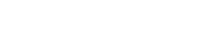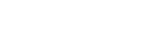# Deep Learning Neural Networks – PART ONE

Jasmin Kurtanović, Software Engineer
12.11.2020.Deep learning, also known as deep structural learning or hierarchical learning, can be considered part of a broader family of machine learning, which is based more on learning the presentation of data, i.e. the characteristics of the data, in addition to performing the task, than just on a specific task-oriented algorithm.

Deep learning models draw inspiration from the flow of information and its processing that takes place in the biological nervous system, specifically from the neural code process, which tries to describe the connection between various stimuli and neural impulses in the brain.

The most well-known deep learning architectures are certainly deep neural networks, DBF (deep belief network), and recurrent neural networks. Some of the fields where deep learning can be applied are computer vision, speech recognition, sound recognition, social filtering network, bioinformatics, drug design, advanced image processing, segmentation, whatever data has a time component, etc. In many scenarios, deep learning has shown equal and even superior results in relation to human expertise.

In short, a deep learning algorithm can be described as a machine learning algorithm that:

• Uses a series of multiple layers that represent nonlinear processing units and serve for data characteristic extraction and transformation. Each of the layers uses the output of the previous layer as its input
• Can use supervised (classification) and unsupervised learning (shape analysis)
• Learns multiple levels of data representation that correspond to different levels of abstraction

As already pointed out, deep learning neural networks can learn useful data characteristics directly from the data. Precisely by combining its layers (which will be explained later), which are interconnected, the neural network uses simple elements that work in parallel, just like the human brain. In this way, deep neural networks are able to make top classification, if they are posed a problem.

Figure 1 shows the basic concept of how this classification operates using a deep neural network. You can see that different layers enter the circulation, so far not so well-known shallow neural networks. There will be more information about them later.

## Convolutional Neural Network Architecture

The deep neural network is trained with large amounts of data, and the architecture of the network itself contains a larger number of neural layers, especially those called convolutional (hence the name convolutional networks). Training such networks is a demanding job in terms of resources and performance, which suggests that this type of processing should be done on the GPU, not the CPU, so that the process is finished in a reasonable time. Figure 2 shows the network training process, i.e. the process of learning data characteristics, in this case images, through iterations and through multiple layers of the network:Figure 2: Architecture and layers of a deep neural network used for classification

Input layer – The input layer of the deep neural network specifies the dimensions and structure of the input data. Specifically, in the case of an image, the height, width and number of channels.

Convolutional layer – The convolutional layer is perhaps the key layer of the deep neural network because it serves to extract data characteristics. This layer has its own parameters such as filter size, number of filters, etc.

Batch normalization layer – This layer normalizes the activations and gradients that propagate through the network, thus making the network optimal for training. It’s mostly used as an intermediate layer between the convolutional and the nonlinearity layers, such as the ReLU layer, in order to speed up the network training process and reduce the network initialization sensitivity.

ReLU layer – The batch normalization layer is usually followed by a layer which represents a nonlinear activation function. The most common activation function is the ReLU (Rectified Linear Unit) function.

Max pooling layer – A pooling layer is a new layer added after the convolutional and the normalization layer. More specifically, after a nonlinearity (e.g. ReLU) has been applied to the feature maps output by a convolutional layer. It is used for surgery reducing the samples, thus reducing the spaciousness of the data characteristics map and removing redundant spatial information. This operation allows you to increase the size of the filter in deep convolutional layers without increasing the number of calculations per layer.

Fully connected layer – Convolutional and data reduction layers are usually followed by one or more fully connected layers. As the name suggests, a fully connected layer is a layer in which the neurons connect to all neurons from the previous layer. This layer combines all possible data characteristics that the previous layers have learned from all parts of the input data and uses them to recognize larger features within the data. The last fully connected layer combines all possible available data characteristics to classify the input data (if there is a problem classification).

Softmax layer – The softmax layer is an activation function that normalizes the output from the fully connected layer. The output of the softmax layer consists of positive numbers whose sum is 1, which can be used as classification probability by the classification layer (which comes the next layer).

Classification layer – The final layer is the classification layer, which takes the output of the softmax layer, assigns mutually exclusive classes for each input and calculates the error, i.e. the probability.

One of the essential functionalities of deep neural networks is the concept of learning transfer.

Learning transfer is the process of using the knowledge of a certain already trained neural network (overtraining) to identify new patterns and new data. Finetuning overtrained neural networks is much faster and easier than training from scratch. By using a pre-trained neural network, it’s possible to learn to train the network on new tasks without defining a new network, and without initial input of a training set that can sometimes be measured with over a million samples. A learning transfer is favorable when there is a small set of new data for network training (for example, less than 1000 images). So, the advantage of an overtrained network is that it already contains the characteristics of the input set – in the case of images, image characteristics – and training is much easier. This concept is shown in Figure 3: